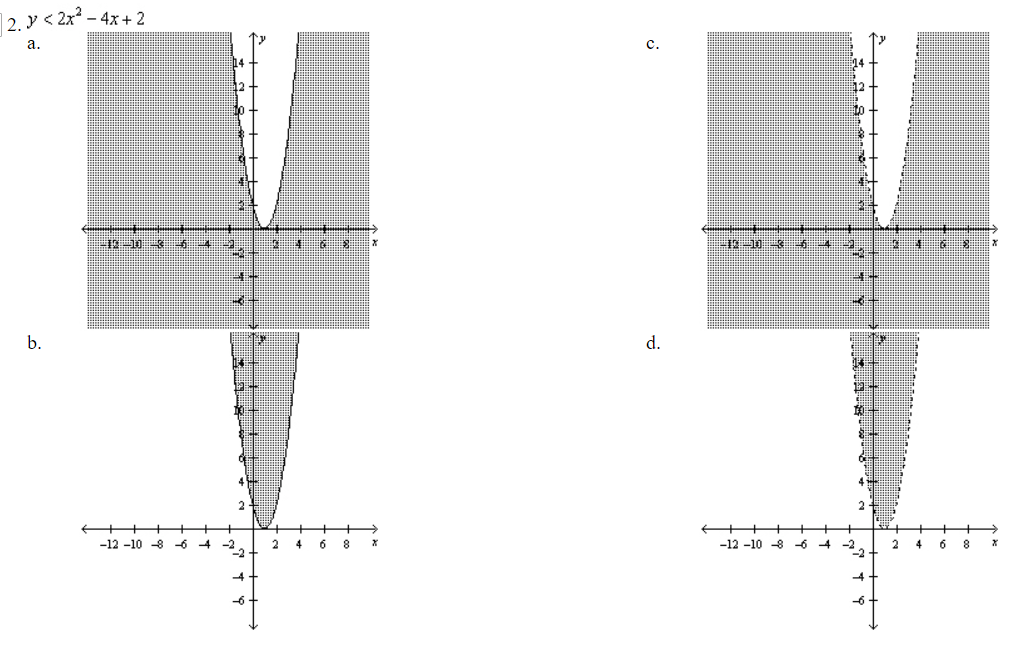# Warmups Topics 7.1

SKIP Number 3Answers (C, skip, B, D  Hint: First, write the related quadratic equation and factor it. Then, use the Zero Product Property and solve each equation. The solution is all real numbers when all test points satisfy the inequality. The solution is an empty set when none of the test points satisfy the inequality. )

(source)